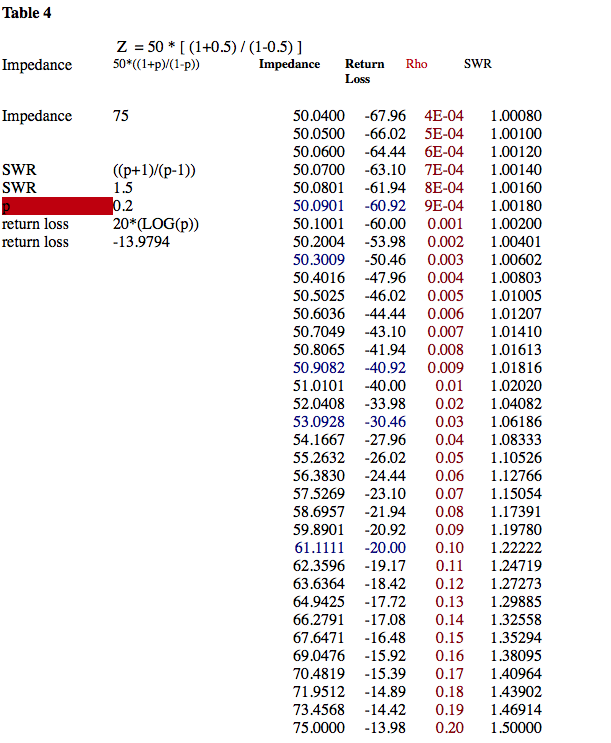D-COAX, INC. • 8201 Jade Tree Ln.
Knoxville, TN 37938  USA
Ph: 503-550-9434 • info@d-coax.com

# Time Domain Reflectometry

Whenever energy is transmitted into a transmission line, the energy may encounter a change in the signal to ground relationship. (An impedance change.) Some of the energy is reflected back toward the source at a different magnitude and time than the original energy effort.

A TDR measures the time that it takes for the energy to return and the size of the energy returning. A TDR makes a very high speed voltage step on a 50W impedance controlled transmission line to the device to be tested. The voltage step will travel into the device and reflect back part of a step when any differences are detected. When the reflected energy magnitude is measured, calculating the difference, the impedance will be known. When the step is sent out and the device is detected, the round trip is calculated for the electrical delay. Velocity of propagation may be derived from knowing the physical length and the electrical length. (The round trip must be divided by 2 to get the one way time.)

TDR instruments make magnitude measurements in Volts and Rho (r).r = (energy reflected)/(energy incident)
If the TDR had a 250mV incident and a 25mV reflection, 100mr = (25mV)/(250mV)
The reflection can be calculated as Reflection = Z* (1+r )/(1-r )
Z = 50W *(1+0.1)/(1-0.1) = 61.1WWhen a circuit board trace has a high enough speed wave traveling on it, transmission line rules must be applied. Current will begin to flow on the surface layer of the conductors (skin effect) and the reactive qualities of the conductors become dominant. The inductance of the center conductor, capacitance of center conductor to ground, resistivity of the center conductor, become important for controlling the optimum transmission of energy.

Z = (L/C)^0.5

When a step is traveling through a transmission line, the step has the effect of charging the capacitance found from the center conductor to ground. When the end of the open circuit transmission line is reached, the charge is 2 times bigger than the incident. The new charge will reflect back down the transmission line and cancel the charge on the next incident step. The TDR will demonstrate this with a short time period and a step up-ward. (No current flow.)

When a 50W resistor is put at the end of the transmission line the current will keep flowing. The TDR will demonstrate this with a short time period and a continuation indefinitely from the end of the transmission line.

If the transmission line had a short circuit on the end, a negative amplitude step reflection would occur and the voltage will also settle to zero.

In many cases, designers add a resistor and capacitor in series to ground to reduce unwanted reflections. (AC coupled Termination) A series resistor on the center conductor only, would do the same job, but it would use a great deal of the incident energy. (DC coupled Termination.)

The system rise time will set the case for what the smallest impedance reflection (discontinuity) can be detected. This system rise time is the combined rise time of the pulse generator, the oscilloscope, the interconnect from the instrumentation to the device, and the device. Use short, high quality, cables to minimize losses because they can begin to cut down the rise time also.

Tr sys = ((Tr gen)+(Tr oscope)+(Tr connect)+(Tr device))^0.5

Back to tutorials page